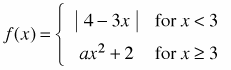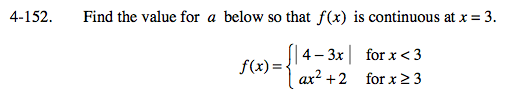### Home > CALC > Chapter 4 > Lesson 4.4.3 > Problem4-152

4-152.
1. Find the value for a below so that f(x) is continuous at x = 3. Homework Help ✎We need to find a value of a such that:

Substitute the left piece of f(x) and the right piece of f(x).

Evaluate the limits:

5 = 9a + 2

Solve for a: